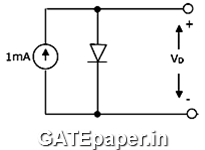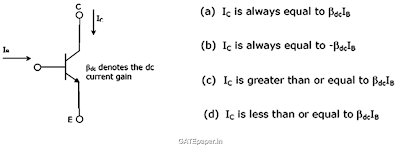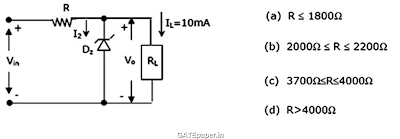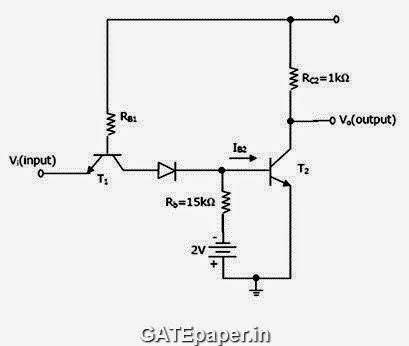### GATE 2002 ECE Video Solutions on EDC (Electronic Devices and Circuits)

1. In the figure shown, a silicon diode is carrying a constant current of 1 mA. When the temperature of the diode is 20oC, diode voltage is found to be 700 mV. If the temperature rises to 40oC, diode voltage becomes approximately equal toa. 740 mV
b. 660 mV
c. 680 mV
d. 700 mV

2. If the transistor in figure is in saturation, then3. A zener diode regulator in figure, is to be designed to meet the specifications : IL = 10 mA, Vo = 10 volts and Vin varies from 30 to 50 volts. The zener diode has VZ = 10 volts and IZK (knee current) = 1 mA. For satisfactory operation4. Consider the following statements in connection with the CMOS inverter in figure, where both the MOSFETs are of enhancement type and both have a threshold voltage of 2 volts.S1: T1 conducts when VI ≥ 2 volts.
S2: T1 is always in saturation when Vo = 0 volts.
Which of the following is correct.
a. Only S1 is TRUE
b. Only S2 is TRUE
c. Both are TRUE
d. Both are FALSE

5. Each transistor in figure, has a dc current gain βdc = 50, cut in voltage Vγ = 0.65 volts and VBEsat = 0.75 volts. The output voltage Vo for T2 in saturation can be as high as 0.2 volts. Assume 0.7 volts drop across a conducting PN junction.Determine
(a) The minimum value IB2 necessary to keep T2 saturation.
(b) The maximum permissible value for the resistance RB1.
(c) The worst case high input (logic 1) and the worst case low input (logic 0)
for which T2 will be either in saturation or in cut off.